# DS博客作业05--查找

| 这个作业属于哪个班级 | 数据结构--网络2011/2012 |
| ---- | ---- | ---- |
| 这个作业的地址 | DS博客作业05--查找 |
| 这个作业的目标 | 学习查找的相关结构 |
| 姓名 | 吴俊豪 |

# 0.PTA得分截图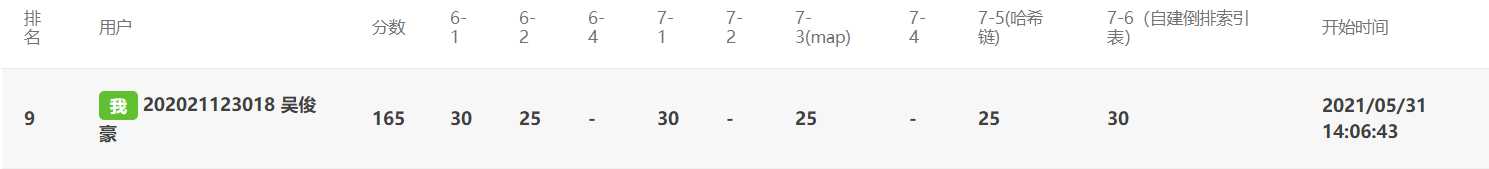# 1.本周学习总结

## 1.1 查找的性能指标

ASL（Average Search Length），即平均查找长度，在查找运算中，由于所费时间在关键字的比较上，所以把平均需要和待查找值比较的关键字次数成为平均查找长度。一个算法的ASL越大，说明时间性能差，反之，时间性能好，这也是显而易见的。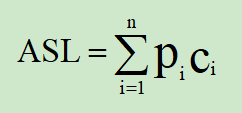## 1.2 静态查找

### 1.2.1 顺序查找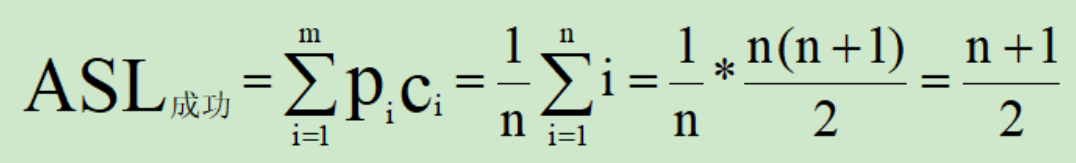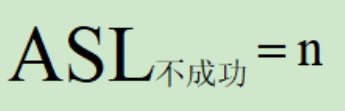### 1.2.2 二分查找(折半查找)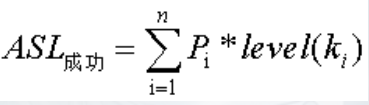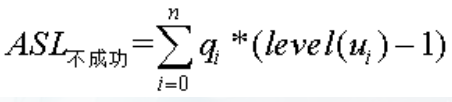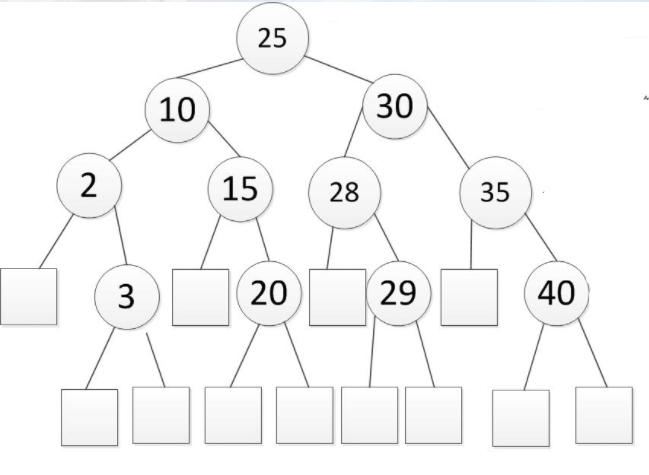ASL(成功)=(1×1+2×2+4×3+3×4)/11=3 (这里的11为查找树的结点个数)
ASL(不成功)=(4×3+8×4)/12=3.67[3.66...] (这里12为该查找树相较完全二叉树的空节点个数)

## 1.3 二叉搜索树

### 1.3.1 如何构建二叉搜索树（操作）

#### 构建

1. 把父节点设置为根节点。
2. 如果新节点内的数据值小于当前节点内的数据值，那么把当前节点设置为当前节点的左孩子。如果新节
点内的数据值大于当前节点内的数据值，那么就将当前节点设为当前节点的右孩子
3. 如果当前节点的左孩子的数值为空，就把新节点插入在这里并且退出循环。否则，跳到 while 循
环的下一次循环操作中。
4. 把当前节点设置为当前节点的右孩子。
5. 如果当前节点的右孩子的数值为空，就把新节点插入在这里并且退出循环。否则，跳到 while 循
环的下一次循环操作中。

### 1.3.2 如何构建二叉搜索树（代码）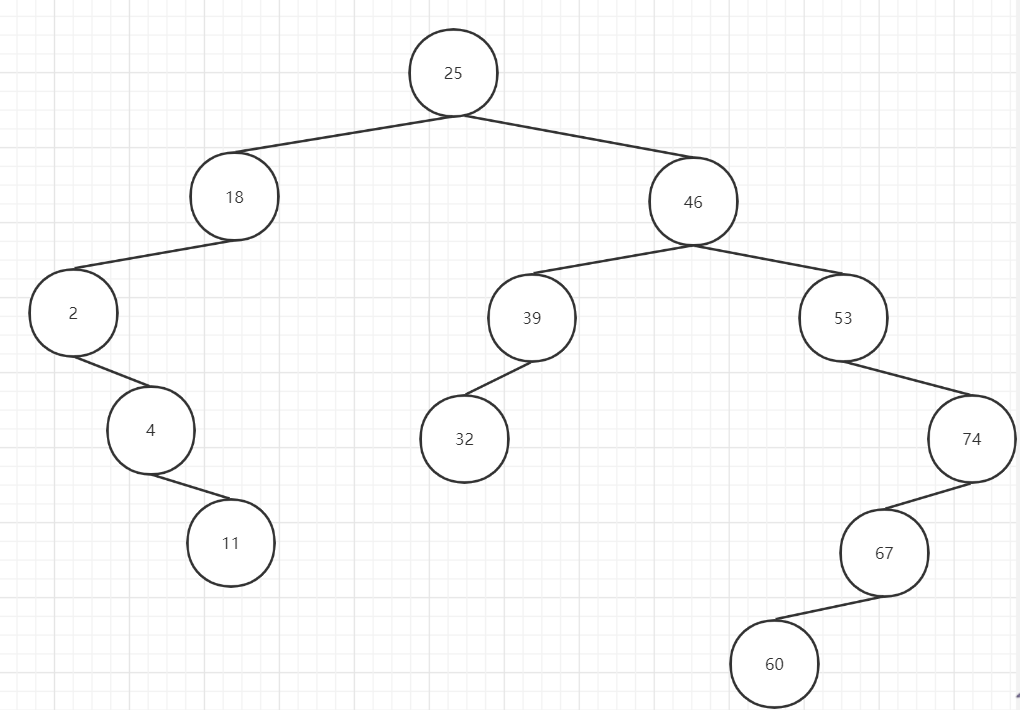ASL成功=1×1+2×2+3×3+3×4+2×5+1×6)/12=3.5
ASL不成功=1×2+3×3+4×4+3×5+2×6)/13=4.15

#### 二叉搜索树的构建

#include<iostream>
#include<fstream>
#include<cmath>
#include<algorithm>
using namespace std;

#define max 100
class Tree {
public:
int a[max];
int last;
};
Tree* T = new Tree; //建立一颗空的二叉搜索树
void CreateBinaryT(int p[], int left, int right, int TRoot);
int main()
{
ifstream sin("a.txt");
int n, i;
int p[max];
while (sin >> n)
{
T->last = n;
for (i = 0; i < n; i++)
sin >> p[i];
sort(p, p + n);
CreateBinaryT(p, 0, n - 1, 0);
for (i = 0; i < T->last; i++)
cout << T->a[i] << " "; //层序输出
cout << endl;
}
return 0;
}

int Getleftson(int n)//左子树结点个数
{
int l, h, i;
for (i = 0; pow(2, i) <= n; i++);
h = i - 1;;
l = n + 1 - pow(2, h);
if (l > pow(2, h - 1))
return pow(2, h) - 1;
else
return n - pow(2, h - 1);
}

void CreateBinaryT(int p[], int left, int right, int TRoot)
{
int n = right - left + 1;
if (n == 0)
return; //递归出口
int l = Getleftson(right - left + 1); //左子树节点个数
T->a[TRoot] = p[left + l];
CreateBinaryT(p, left, left + l - 1, TRoot * 2 + 1);
CreateBinaryT(p, left + l + 1, right, TRoot * 2 + 2);
}


int InsertBST(BSTree &p,KeyType k)
{
if (p==NULL)//原树为空
{
p=new BSTNode;
p->key=k;
p->lchild=p->rchild=NULL;
return 1;
}
else if  (k==p->key) //相同关键字的节点0
return 0;
else if (k<p->key)
return InsertBST(p->lchild,k);//插入到左子树
else
return InsertBST(p->rchild,k);//插入到右子树
}


#### 二叉搜索树的删除

void deleteBst(TreeNode** r, TreeNode* P)
{
TreeNode* t = *r;
TreeNode* parent = getParentBst(t, P);
TreeNode* minRight; //找到右节点为树的最小节点
if (*r == NULL || P == NULL)
return;
if (P->_left == NULL && P->_right == NULL) //第一种情况
{
if (*r == P)
{
free(t);
*r = NULL;
return;
}
if (parent->_left == P)
parent->_left = NULL;
else
parent->_right = NULL;
free(P);
return;
}
else if (P->_left != NULL && P->_right == NULL)//第二种
{
if (*r == P)
{
*r = P->_left;
free(P);
return;
}
if (parent->_left == P)
parent->_left = P->_left;
else
parent->_right = P->_left;
free(P);
return;
}
else if (P->_left == NULL && P->_right != NULL)//第二种
{
if (*r == P)
{
*r = P->_right;
free(P);
return;
}
if (parent->_left == P)
parent->_left = P->_right;
else
parent->_right = P->_right;
free(P);
return;
}
else
{
minRight = getMinBst(P->_right);
P->_data = minRight->_data;
parent = getParentBst(t, minRight);

if (minRight == P->_right)
parent->_right = minRight->_right;  //这里P的_right无论是否为空都可以
else
parent->_left = minRight->_right;
free(minRight);
}


## 1.4 AVL树

### 1.4.1 AVL树解决什么问题，其特点是什么？

AVL树本质上还是一棵二叉搜索树，它的特点是:

1. 本身首先是一棵二叉搜索树.
2. 带有平衡条件:每个结点的左右子树的高度之差的绝对值(平衡因子)最多为1.
也就是说，AVL树，本质上是带了平衡功能的二叉查找树(二叉排序树，二叉搜索树).

### 1.4.2 结合一组数组，介绍AVL树的4种调整做法。

AVL树在保证自身平衡的时候需要四种调整方法,分别是RR,LL,RL,LR类型
RR: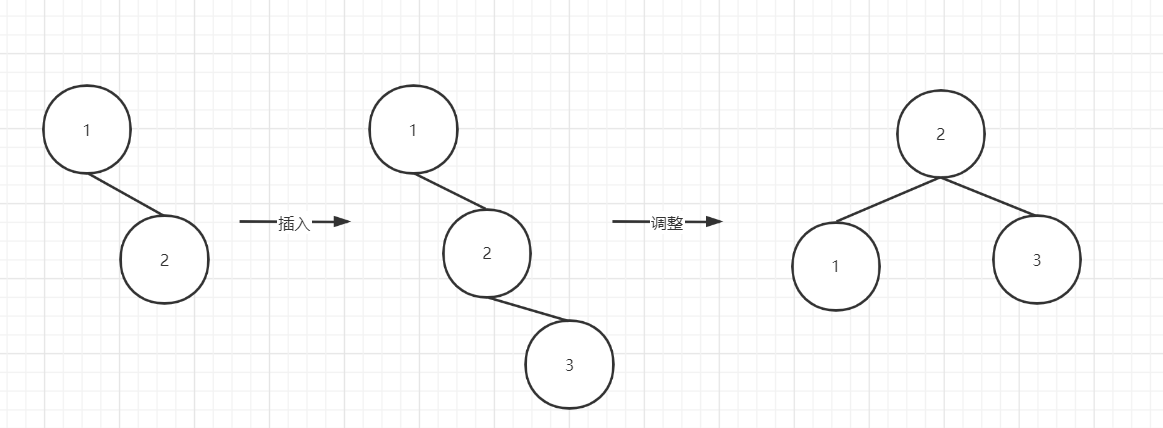LL: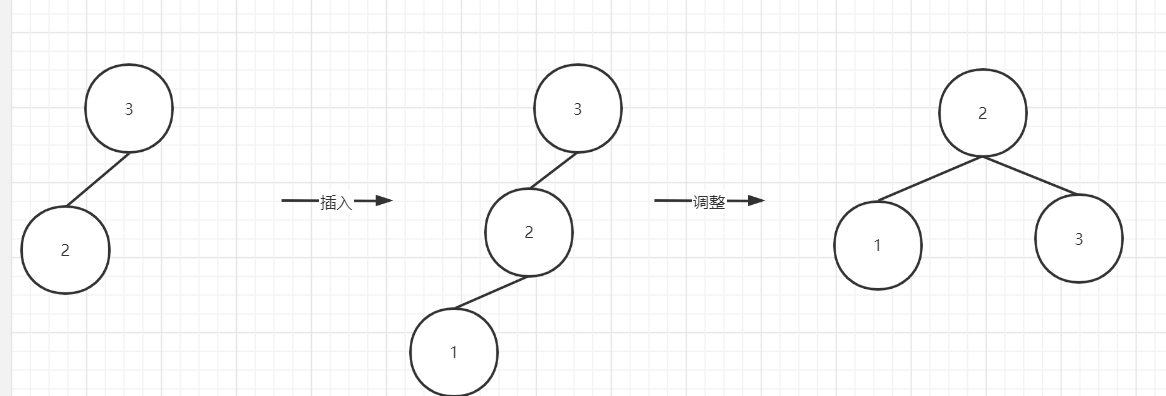RL: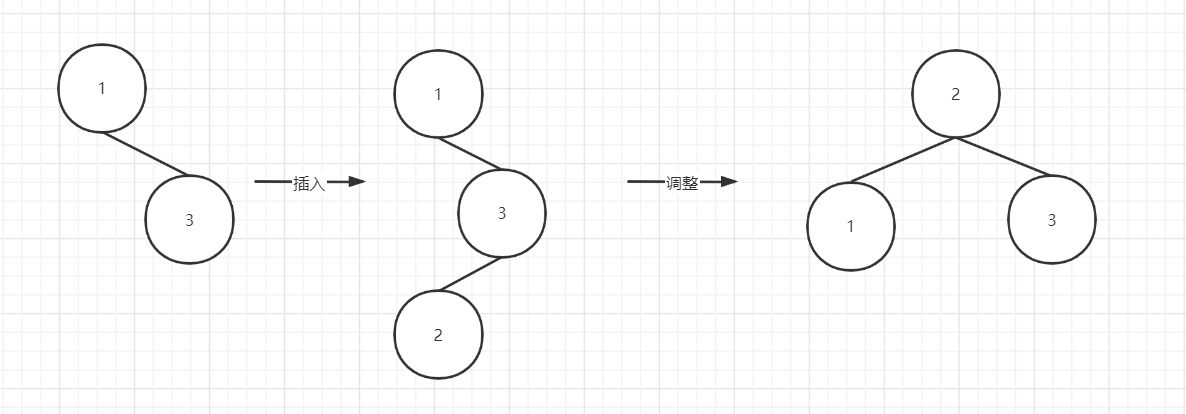LR: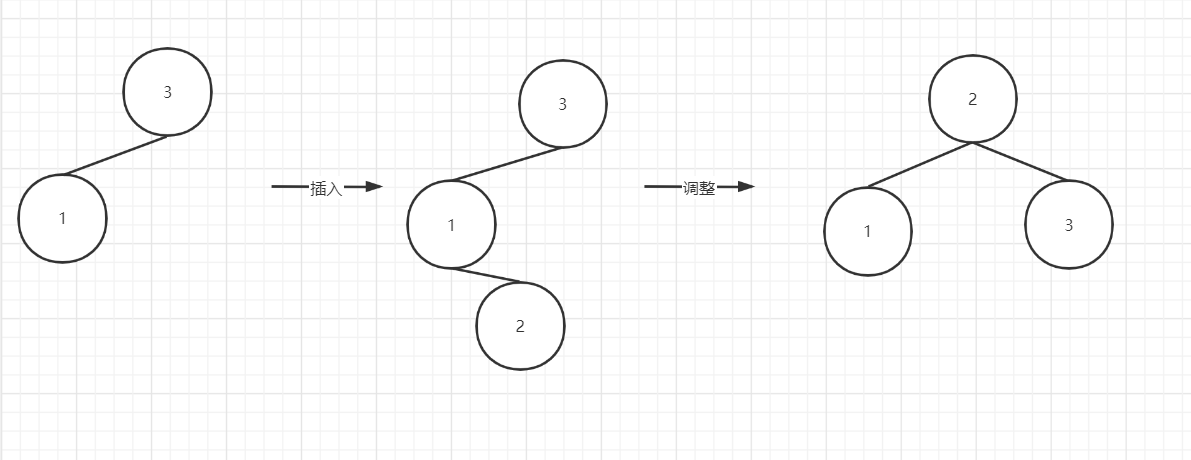h=log2（n+1）

#### 介绍基于AVL树结构实现的STL容器map的特点、用法。

Map是STL的一个关联容器，翻译为映射，数组也是一种映射。如：int a 是int 到 int的映射，而a=25,是把5映射到25。数组总是将int类型映射到其他类型。这带来一个问题，有时候希望把string映射成一个int ，数组就不方便了，这时就可以使用map。map可以将任何基本类型（包括STL容器）映射到任何基本类型（包括STL容器）。

begin() 返回指向map头部的迭代器
end() 返回指向map末尾的迭代器
rbegin() 返回一个指向map尾部的逆向迭代器
rend() 返回一个指向map头部的逆向迭代器
lower_bound() 返回键值>=给定元素的第一个位置
upper_bound() 返回键值>给定元素的第一个位置
empty() 如果map为空则返回true
max_size() 返回可以容纳的最大元素个数
size() 返回map中元素的个数
clear(） 删除所有元素
count() 返回指定元素出现的次数
equal_range() 返回特殊条目的迭代器对
erase() 删除一个元素
swap() 交换两个map
find() 查找一个元素
get_allocator() 返回map的配置器
insert() 插入元素
key_comp() 返回比较元素key的函数
value_comp() 返回比较元素value的函数

## 1.5 B-树和B+树

### B-树定义。结合数据介绍B-树的插入、删除的操作，尤其是节点的合并、分裂的情况

#### 一颗m阶的B树定义如下：

1）每个结点最多有m-1个关键字。
2）根结点最少可以只有1个关键字。
3）非根结点至少有Math.ceil(m/2)-1个关键字。
4）每个结点中的关键字都按照从小到大的顺序排列，每个关键字的左子树中的所有关键字都小于它，而右子树中的所有关键字都大于它。
5）所有叶子结点都位于同一层，或者说根结点到每个叶子结点的长度都相同。

#### 插入操作

1）根据要插入的key的值，找到叶子结点并插入。
2）判断当前结点key的个数是否小于等于m-1，若满足则结束，否则进行第3步。
3）以结点中间的key为中心分裂成左右两部分，然后将这个中间的key插入到父结点中，这个key的左子树指向分裂后的左半部分，这个key的右子支指向分裂后的右半部分，然后将当前结点指向父结点，继续进行第3步。

#### 删除操作

1）如果当前需要删除的key位于非叶子结点上，则用后继key（这里的后继key均指后继记录的意思）覆盖要删除的key，然后在后继key所在的子支中删除该后继key。此时后继key一定位于叶子结点上，这个过程和二叉搜索树删除结点的方式类似。删除这个记录后执行第2步
2）该结点key个数大于等于Math.ceil(m/2)-1，结束删除操作，否则执行第3步。
3）如果兄弟结点key个数大于Math.ceil(m/2)-1，则父结点中的key下移到该结点，兄弟结点中的一个key上移，删除操作结束。

### B+树定义，其要解决问题

#### 定义

1）B+树包含2种类型的结点：内部结点（也称索引结点）和叶子结点。根结点本身即可以是内部结点，也可以是叶子结点。根结点的关键字个数最少可以只有1个。
2）B+树与B树最大的不同是内部结点不保存数据，只用于索引，所有数据（或者说记录）都保存在叶子结点中。
3） m阶B+树表示了内部结点最多有m-1个关键字（或者说内部结点最多有m个子树），阶数m同时限制了叶子结点最多存储m-1个记录。
4）内部结点中的key都按照从小到大的顺序排列，对于内部结点中的一个key，左树中的所有key都小于它，右子树中的key都大于等于它。叶子结点中的记录也按照key的大小排列。
5）每个叶子结点都存有相邻叶子结点的指针，叶子结点本身依关键字的大小自小而大顺序链接。

## 1.6 散列查找

### 1.6.1 哈希表的设计主要涉及哪几个内容？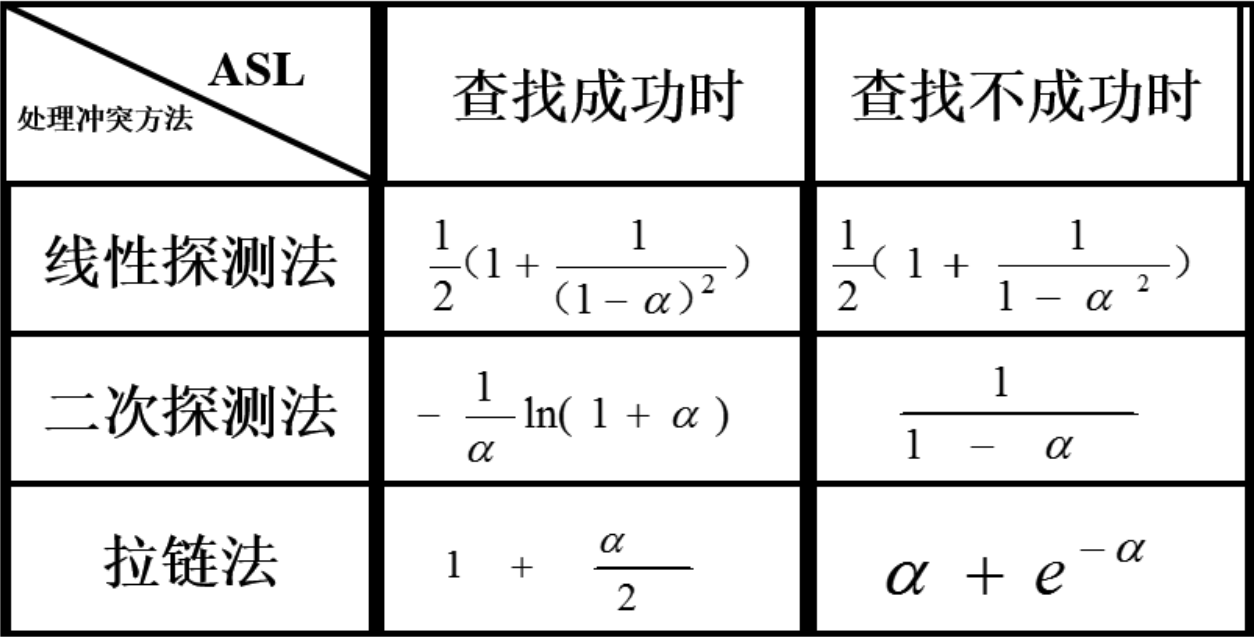### 1.6.2 结合数据介绍哈希表的构造及ASL成功、不成功的计算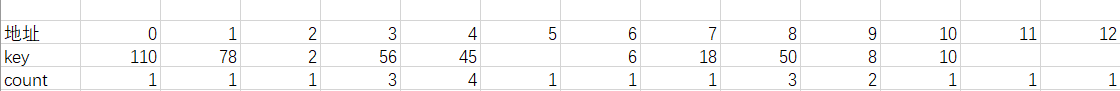ASL(成功)=(1×9+2+3×2+4)/10=1.8
ASL(不成功)=(6+5+4+3+2+1+6+5+4+3+2)/11=41/11

### 1.6.3 结合数据介绍哈希链的构造及ASL成功、不成功的计算ASL(成功)=15+21)/6=7/6=1.17
ASL(不成功)=0+1+0+1+2+1+1)/7=6/7=0.86

# 2.PTA题目介绍

## 2.1 是否完全二叉搜索树

### 2.1.1 解题思路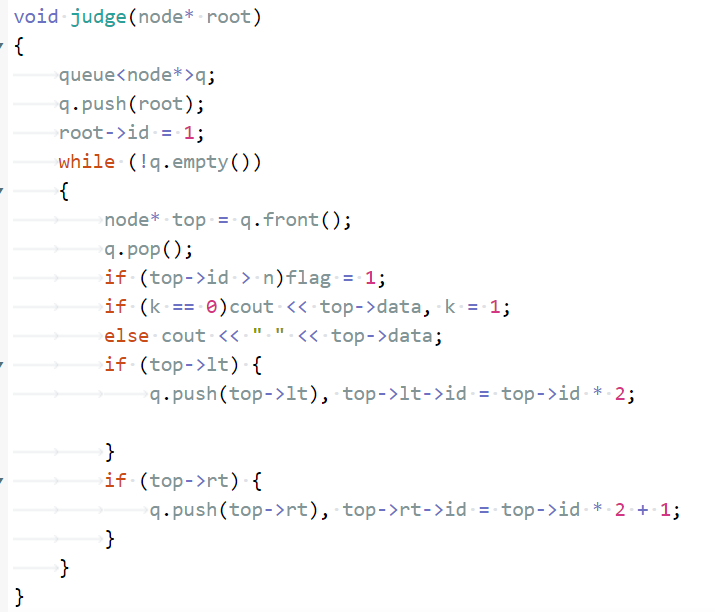### 2.1.2 提交列表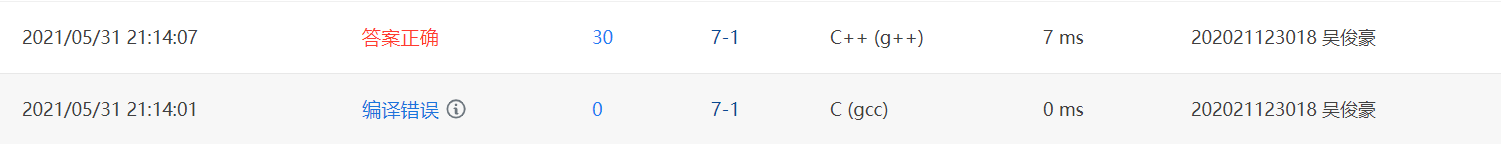## 2.2 航空公司VIP客户查询

### 2.2.1 解题思路

（1）根据输入的人数，初始化一个哈希表，哈希表中包括表的长度和表的元素结点的地址数组（用于将下标匹配相应的哈希值）
（2）循环输入n个人的身份证号码和里程，进行判断，
1、先将此身份证号通过相应的算法得到哈希值
2、如果这个人是第一次记录，则创造新的结点存储信息，如果已经存在了该人，直接累加里程
3、在计算某个身份证对应的地址时，先计算相应的哈希值得到其在地址数字中的下标，当此下标对应的地址的下一个结点存在且下一个结点存储的id与当前不同时，将指针后移，这样就能防止哈希表的冲突出现
（3）根据输入的身份证号查询相关里程

### 2.2.2 提交列表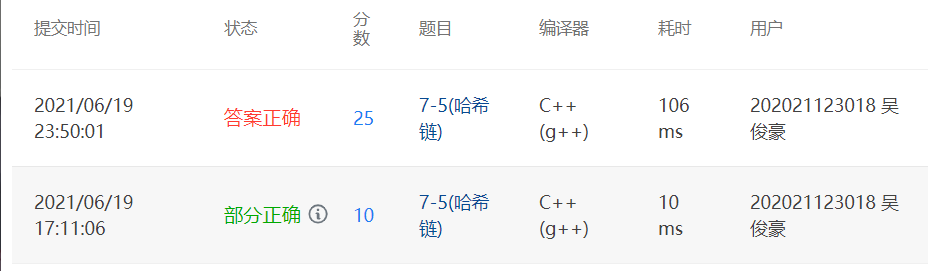## 2.3 基于词频的文件相似度

### 2.3.1 解题思路

1、存储：用一张哈希表存储单词以及对应所在的文件，再用一张文件表，存储每个文件的词汇量以及单词在哈希表中的位置
2、查询：先在文件表中查询对应的文件名，（取文件词汇量较少的文件名）-> 找到对应文件名中的词汇所在位置-> 根据此单词的位置到哈希表中查找单词所在文件列表->从而判断该单词是否是两文件的公共词汇

### 2.3.2 提交列表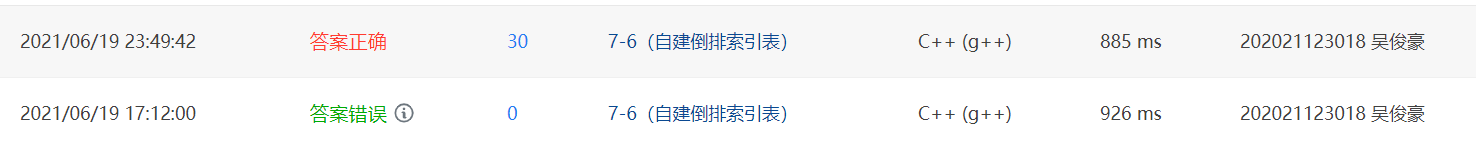### 2.3.3 本题知识点

posted @ 2021-06-14 09:53  Qurare  阅读(129)  评论(0编辑  收藏  举报